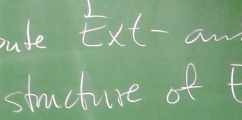BIREP – Representations of finite dimensional algebras at Bielefeld# Quantisation spaces of cluster algebras

This site is intended as an appendum to the paper "Quantisation spaces of cluster algebras", joint with Philipp Lampe. It can be found here on the arXiv.
Please note that this site was created using mathJax and the Sage Cell Server. In case you experience issues either with the displayed LaTeX or no computing cells appear, feel free to contact me. General compatibility requirements can be found here and here for mathJax and the Sage Cell Server respectively.

The precise definition of quantum cluster algebras can be found in the aforementioned acticle or the initial paper from Berenstein and Zelevinksy. Thus we focus on our main constructions outlined below.

An initial cluster seed of a given cluster algebra carries, along with its cluster variables, an $m\times n$ -matrix $\tilde{B}=\left[\begin{smallmatrix}B\\C\\\end{smallmatrix}\right]$ with the $n\times n$ principal part $B$. By definition, a skew-symmetric $m\times m$ integer matrix $\Lambda = (\lambda_{i,j})$ is called compatible to $\tilde{B}$ if there exists a diagonal $n\times n$ matrix $D'=\textrm{diag}(d'_1,d'_2,\ldots,d'_n)$ with positive integers $d'_1,d'_2,\ldots,d'_n$ such that $\tilde{B}^T\Lambda =\left[\begin{matrix}D' & 0 \end{matrix}\right]$ as a $n\times n$ plus $n\times (m-n)$ block matrix.
Our paper then discusses the question, "When does a quantisation for a given cluster algebra $\mathcal{A}(\tilde{B})$ exist and how unique is it?"

The existence of a compatible matrix pair $(\tilde{B}, \Lambda)$ depends on the rank of $\tilde{B}$: if $\mathrm{rk}(\tilde{B}) < n$, then no quantisation exists. Otherwise, one can construct a compatible $\Lambda$ by viewing the columns of $\tilde{B}$ as vectors, completing their set to a basis of $\mathbb{Q}^m$ and obtaining the following result.
Theorem: Let $D$ be a skew-symmetriser of $B$. There exists a skew-symmetric $m\times m$-matrix $\Lambda$ with integer coefficients and a multiple $D'=\lambda D$ with $\lambda\in\mathbb{Q}^{+}$ such that $\tilde{B}^T\Lambda =\left[\begin{matrix}D'&0\end{matrix}\right]$.

We illustrate the construction using Sage, details on the relationship between the choice of added basis vectors and $\Lambda$ can be found in the article.

After loading the new function, we can now compute a $\Lambda$ from the theorem above.

The construction of $\Lambda$ above depends on a choice of basis completion. This ambiguity we reformulate by giving a generating set of integer matrices for the equation $\tilde{B}^T\Lambda = \left[\begin{matrix}0 &0\end{matrix}\right].$ The stated dependency does not occur for $0$ or $1$ frozen vertices, hence we start with the case for $m=n+2$. We only provide the Sage functions at this point, the general construction can be found in the paper.

Let us check, that what we did so far has worked out. First, we are interested whether $B^T \cdot \mathrm{minorBlock}(B)$ equals the zero matrix. The second entry of the list gives the product $B^T \cdot \mathrm{inhomSolution}(B)$:

Next, if a given $\tilde{B}$ is associated to a quiver with more than two frozen vertices, we need to use the minor blocks and compose them. This can be achieved via the following code, see Section 4.2 in the paper.

Again, after loading these definitions, we can compute the composed minor blocks and retrieve a list of matrices satisfying the equation $\tilde{B}^T\Lambda = \left[\begin{smallmatrix}0 &0\end{smallmatrix}\right]$:

Lastly, we can again check the correctness of our computations. For each element in the list of results returned from compBlock, compare with the appropriate 0-matrix.

Note that all cells with an 'Evaluate'-button underneath can be altered - Feel free to test the functions on skew-symmetrisable matrices of your own choosing. Please feel free to contact me for comments and/or questions regarding our results or how we used Sage in the process of developing our findings.